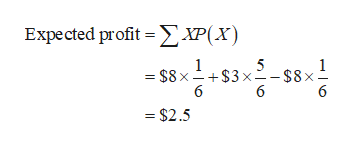# Suppose that you are offered the following "deal." You roll a six sided die. If you roll a 6, you win \$8. If you roll a 2, 3, 4 or 5, you win \$3. Otherwise, you pay \$8. a. Complete the PDF Table. List the X values, where X is the profit, from smallest to largest. Round to 4 decimal places where appropriate. Probability Distribution TableXP(X)      b. Find the expected profit. \$ (Round to the nearest cent) c. Interpret the expected value.If you play many games, on average, you will likely win, or lose if negative, close to this amount.You will win this much if you play a game.This is the most likely amount of money you will win.

Question
39 views

Suppose that you are offered the following "deal." You roll a six sided die. If you roll a 6, you win \$8. If you roll a 2, 3, 4 or 5, you win \$3. Otherwise, you pay \$8.

a. Complete the PDF Table. List the X values, where X is the profit, from smallest to largest. Round to 4 decimal places where appropriate.

Probability Distribution Table

X P(X)

b. Find the expected profit. \$ (Round to the nearest cent)

c. Interpret the expected value.

• If you play many games, on average, you will likely win, or lose if negative, close to this amount.
• You will win this much if you play a game.
• This is the most likely amount of money you will win.
check_circle

Step 1

a.

The possible outcomes when rolling a die is {1, 2, 3, 4, 5 and 6}.

The formula for finding probability of an event is shown below:

Step 2

Using the given information, the probability distribution table is obtained as follows:

Step 3

b)

The expected profit is obt...help_outlineImage TranscriptioncloseExpected profit XP(x) 1 \$3 x--\$8x- 6 1 = \$8 x 6 6 - \$2.5 fullscreen

### Want to see the full answer?

See Solution

#### Want to see this answer and more?

Solutions are written by subject experts who are available 24/7. Questions are typically answered within 1 hour.*

See Solution
*Response times may vary by subject and question.
Tagged in

### Statistics